Projectile Motion

Learning Objectives

By the end of this section, you will be able to:

• Identify and explain the properties of a projectile, such as acceleration due to gravity, range, maximum height, and trajectory.
• Determine the location and velocity of a projectile at different points in its trajectory.
• Apply the principle of independence of motion to solve projectile motion problems.

Projectile motion is the motion of an object thrown or projected into the air, subject to only the acceleration of gravity. The object is called a projectile, and its path is called its trajectory. The motion of falling objects, as covered in Problem-Solving Basics for One-Dimensional Kinematics, is a simple one-dimensional type of projectile motion in which there is no horizontal movement. In this section, we consider two-dimensional projectile motion, such as that of a football or other object for which air resistance is negligible.

The most important fact to remember here is that motions along perpendicular axes are independent and thus can be analyzed separately. This fact was discussed in Kinematics in Two Dimensions: An Introduction, where vertical and horizontal motions were seen to be independent. The key to analyzing two-dimensional projectile motion is to break it into two motions, one along the horizontal axis and the other along the vertical. (This choice of axes is the most sensible, because acceleration due to gravity is vertical—thus, there will be no acceleration along the horizontal axis when air resistance is negligible.) As is customary, we call the horizontal axis the x-axis and the vertical axis the y-axis. Figure 1 illustrates the notation for displacement, where s is defined to be the total displacement and x and y are its components along the horizontal and vertical axes, respectively. The magnitudes of these vectors are s, x, and y. (Note that in the last section we used the notation A to represent a vector with components Ax and Ay. If we continued this format, we would call displacement s with components sx and sy. However, to simplify the notation, we will simply represent the component vectors as x and y.)

Of course, to describe motion we must deal with velocity and acceleration, as well as with displacement. We must find their components along the x– and y-axes, too. We will assume all forces except gravity (such as air resistance and friction, for example) are negligible. The components of acceleration are then very simple: a= –= –9.80 m/s2. (Note that this definition assumes that the upwards direction is defined as the positive direction. If you arrange the coordinate system instead such that the downwards direction is positive, then acceleration due to gravity takes a positive value.) Because gravity is vertical, ax=0. Both accelerations are constant, so the kinematic equations can be used.

Review of Kinematic Equations (constant a)

$x={x}_{0}+\bar{v}t\\$
$\bar{v}=\frac{{v}_{0}+v}{2}\\$
$v={v}_{0}+\text{at}\\$
$x={x}_{0}+{v}_{0}t+\frac{1}{2}{\text{at}}^{2}\\$
${v}^{2}={{v}_{0}}^{2}+2a\left(x-{x}_{0}\right)\\$.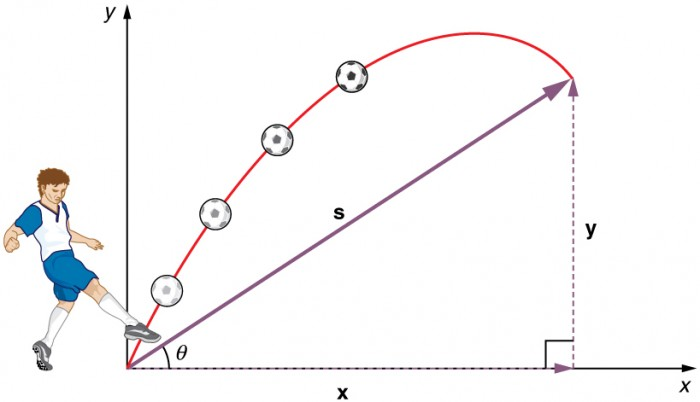Figure 1. The total displacement s of a soccer ball at a point along its path. The vector s has components x and y along the horizontal and vertical axes. Its magnitude is s, and it makes an angle θ with the horizontal.

Given these assumptions, the following steps are then used to analyze projectile motion:

Step 1. Resolve or break the motion into horizontal and vertical components along the x- and y-axes. These axes are perpendicular, so Ax = A cos θ and Ay = A sin θ are used. The magnitude of the components of displacement s along these axes are x and y. The magnitudes of the components of the velocity v are Vx = V cos θ and Vy = v sin θ where v is the magnitude of the velocity and θ is its direction, as shown in 2. Initial values are denoted with a subscript 0, as usual.

Step 2.Treat the motion as two independent one-dimensional motions, one horizontal and the other vertical. The kinematic equations for horizontal and vertical motion take the following forms:

Horizontal Motion (ax = 0)
x = x0 + vxt
vv0v= velocity is a constant.
Vertical Motion (assuming positive is up ay = -g = -9.8 m/s2)
$y={y}_{0}+\frac{1}{2}\left({v}_{0y}+{v}_{y}\right)t\\$
${v}_{y}={v}_{0y}-\text{gt}\\$
$y={y}_{0}+{v}_{0y}t-\frac{1}{2}{\mathrm{gt}}^{2}\\$
${{v}_{y}}^{2}={{v}_{0y}}^{2}-2g\left(y-{y}_{0}\right)\\$.

Step 3. Solve for the unknowns in the two separate motions—one horizontal and one vertical. Note that the only common variable between the motions is time t. The problem solving procedures here are the same as for one-dimensional kinematics and are illustrated in the solved examples below.

Step 4. Recombine the two motions to find the total displacement s and velocity v. Because the x – and y -motions are perpendicular, we determine these vectors by using the techniques outlined in the Vector Addition and Subtraction: Analytical Methods and employing $A=\sqrt{{{A}_{x}}^{2}+{{A}_{y}}^{2}}\\$ and θ = tan−1 (Ay/Ax) in the following form, where θ is the direction of the displacement s and θv is the direction of the velocity v:

Total displacement and velocity

$s=\sqrt{{x}^{2}+{y}^{2}}\\$
θ = tan−1 (y/x)
$v=\sqrt{{{v}_{x}}^{2}+{{v}_{y}}^{2}}\\$
θ= tan−1 (vy/vx).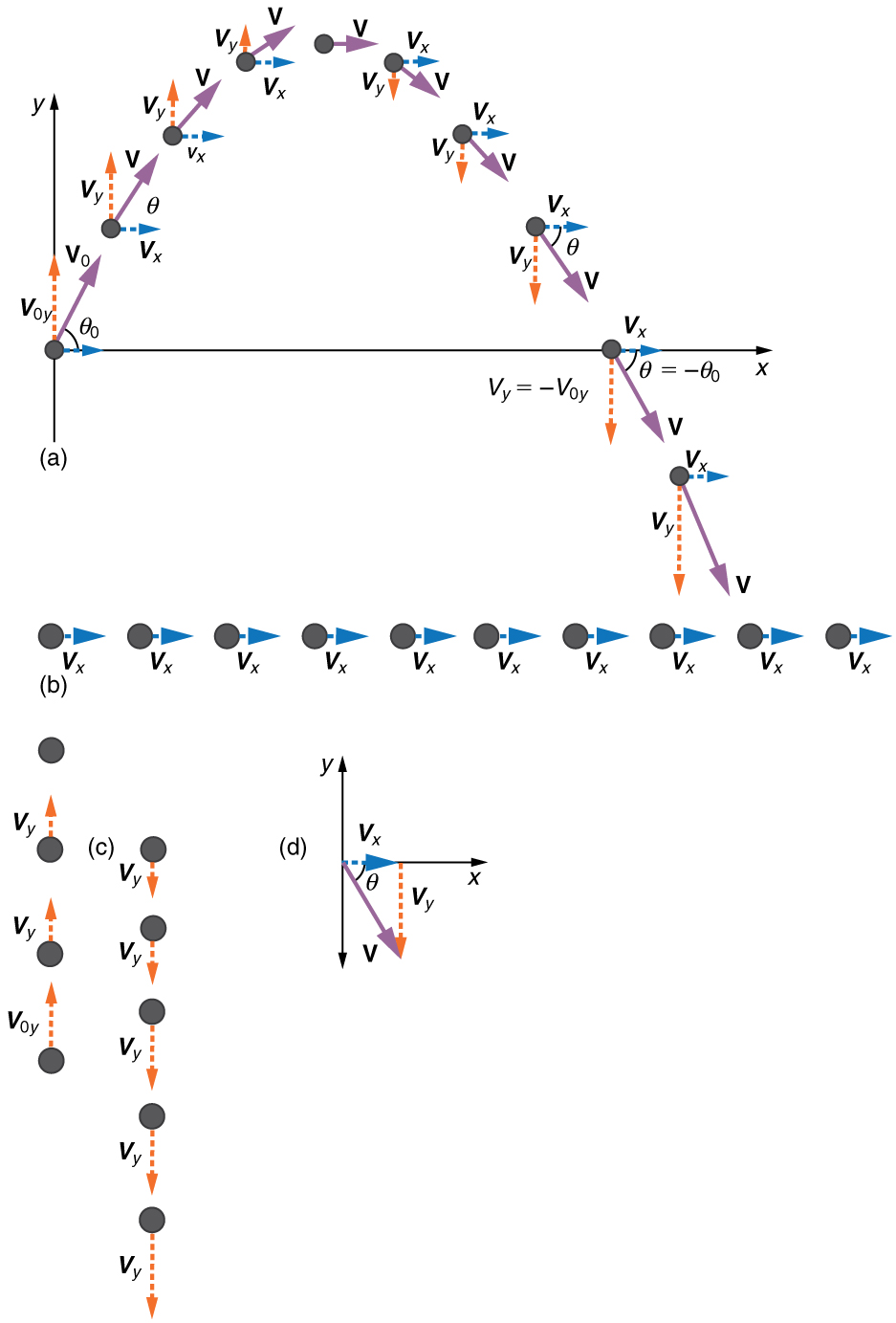Figure 2. (a) We analyze two-dimensional projectile motion by breaking it into two independent one-dimensional motions along the vertical and horizontal axes. (b) The horizontal motion is simple, because ax=0 and vx is thus constant. (c) The velocity in the vertical direction begins to decrease as the object rises; at its highest point, the vertical velocity is zero. As the object falls towards the Earth again, the vertical velocity increases again in magnitude but points in the opposite direction to the initial vertical velocity. (d) The x – and y -motions are recombined to give the total velocity at any given point on the trajectory.

Example 1. A Fireworks Projectile Explodes High and Away

During a fireworks display, a shell is shot into the air with an initial speed of 70.0 m/s at an angle of 75.0º above the horizontal, as illustrated in Figure 3. The fuse is timed to ignite the shell just as it reaches its highest point above the ground. (a) Calculate the height at which the shell explodes. (b) How much time passed between the launch of the shell and the explosion? (c) What is the horizontal displacement of the shell when it explodes?

Strategy

Because air resistance is negligible for the unexploded shell, the analysis method outlined above can be used. The motion can be broken into horizontal and vertical motions in which a= 0 and a= –g. We can then define x0 and y0 to be zero and solve for the desired quantities.

Solution for (a)

By “height” we mean the altitude or vertical position y above the starting point. The highest point in any trajectory, called the apex, is reached when vy=0. Since we know the initial and final velocities as well as the initial position, we use the following equation to find y: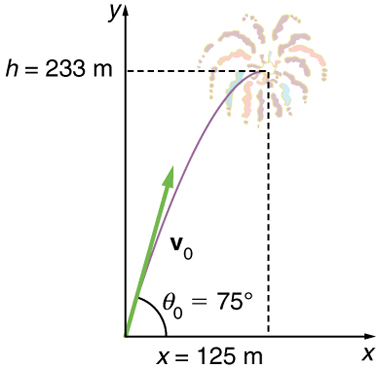Figure 3. The trajectory of a fireworks shell. The fuse is set to explode the shell at the highest point in its trajectory, which is found to be at a height of 233 m and 125 m away horizontally.

Because y0 and vy are both zero, the equation simplifies to

0 = vOy2 – 2gy.

Solving for y gives

$y=\frac{{{v}_{0y}}^{2}}{2g}\\$.

Now we must find v0y, the component of the initial velocity in the y-direction. It is given by v0y = v0 sin θ, where v0y is the initial velocity of 70.0 m/s, and θ= 75.0º is the initial angle. Thus,

vOy = v0 sin θ0 = (70.0 m/s)(sin 75º) = 67.6 m/s

and y is

$y=\frac{\left(67.6\text{ m/s}\right)^{2}}{2\left(9.80\text{ m/s}^{2}\right)}\\$,

so that

= 233 m.

Discussion for (a)

Note that because up is positive, the initial velocity is positive, as is the maximum height, but the acceleration due to gravity is negative. Note also that the maximum height depends only on the vertical component of the initial velocity, so that any projectile with a 67.6 m/s initial vertical component of velocity will reach a maximum height of 233 m (neglecting air resistance). The numbers in this example are reasonable for large fireworks displays, the shells of which do reach such heights before exploding. In practice, air resistance is not completely negligible, and so the initial velocity would have to be somewhat larger than that given to reach the same height.

Solution for (b)

As in many physics problems, there is more than one way to solve for the time to the highest point. In this case, the easiest method is to use $y={y}_{0}+\frac{1}{2}\left({v}_{0y}+{v}_{y}\right)t\\$. Because y0 is zero, this equation reduces to simply

$y=\frac{1}{2}\left({v}_{0y}+{v}_{y}\right)t\\$.

Note that the final vertical velocity, vy, at the highest point is zero. Thus,

$\begin{array}{lll}t& =& \frac{2y}{\left({v}_{0y}+{v}_{y}\right)}=\frac{2\left(\text{233 m}\right)}{\left(\text{67.6 m/s}\right)}\\ & =& 6.90\text{ s}\end{array}\\$.

Discussion for (b)

This time is also reasonable for large fireworks. When you are able to see the launch of fireworks, you will notice several seconds pass before the shell explodes. (Another way of finding the time is by using $y={y}_{0}+{v}_{0y}t-\frac{1}{2}{\text{gt}}^{2}\\$, and solving the quadratic equation for t.)

Solution for (c)

Because air resistance is negligible, ax=0 and the horizontal velocity is constant, as discussed above. The horizontal displacement is horizontal velocity multiplied by time as given by xvxt, where x0 is equal to zero:

vxt,

where vx is the x-component of the velocity, which is given by vx = v0 cos θ0 Now,

vx = v0 cos θ0 = (70.0 m/s)(cos 75º) = 18.1 m/s

The time t for both motions is the same, and so x is

x = (18.1 m/s)(6.90 s) = 125 m.

Discussion for (c)

The horizontal motion is a constant velocity in the absence of air resistance. The horizontal displacement found here could be useful in keeping the fireworks fragments from falling on spectators. Once the shell explodes, air resistance has a major effect, and many fragments will land directly below.

In solving part (a) of the preceding example, the expression we found for y is valid for any projectile motion where air resistance is negligible. Call the maximum height y=h; then,

$h=\frac{{{v}_{0y}}^{2}}{2g}\\$.

This equation defines the maximum height of a projectile and depends only on the vertical component of the initial velocity.

Defining a Coordinate System

It is important to set up a coordinate system when analyzing projectile motion. One part of defining the coordinate system is to define an origin for the x and y positions. Often, it is convenient to choose the initial position of the object as the origin such that x= 0 and y= 0. It is also important to define the positive and negative directions in the x and y directions. Typically, we define the positive vertical direction as upwards, and the positive horizontal direction is usually the direction of the object’s motion. When this is the case, the vertical acceleration, g, takes a negative value (since it is directed downwards towards the Earth). However, it is occasionally useful to define the coordinates differently. For example, if you are analyzing the motion of a ball thrown downwards from the top of a cliff, it may make sense to define the positive direction downwards since the motion of the ball is solely in the downwards direction. If this is the case, g takes a positive value.

Example 2. Calculating Projectile Motion: Hot Rock Projectile

Kilauea in Hawaii is the world’s most continuously active volcano. Very active volcanoes characteristically eject red-hot rocks and lava rather than smoke and ash. Suppose a large rock is ejected from the volcano with a speed of 25.0 m/s and at an angle 35.0º above the horizontal, as shown in Figure 4. The rock strikes the side of the volcano at an altitude 20.0 m lower than its starting point. (a) Calculate the time it takes the rock to follow this path. (b) What are the magnitude and direction of the rock’s velocity at impact?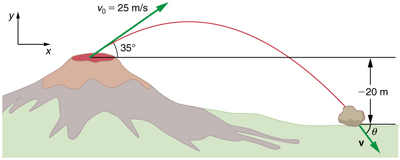Figure 4. The trajectory of a rock ejected from the Kilauea volcano.

Strategy

Again, resolving this two-dimensional motion into two independent one-dimensional motions will allow us to solve for the desired quantities. The time a projectile is in the air is governed by its vertical motion alone. We will solve for t first. While the rock is rising and falling vertically, the horizontal motion continues at a constant velocity. This example asks for the final velocity. Thus, the vertical and horizontal results will be recombined to obtain v and θv at the final time t determined in the first part of the example.

Solution for (a)

While the rock is in the air, it rises and then falls to a final position 20.0 m lower than its starting altitude. We can find the time for this by using

$y={y}_{0}+{v}_{0y}t-\frac{1}{2}{\text{gt}}^{2}\\$.

If we take the initial position y0 to be zero, then the final position is = −20.0 m. Now the initial vertical velocity is the vertical component of the initial velocity, found from vOy = v0 sin θ0 = (25.0 m/s)(sin 35.0º) = 14.3 m/s. Substituting known values yields

−20.0 m = (14.3 m/s)− (4.90 m/s2)t2.

Rearranging terms gives a quadratic equation in t:

(4.90 m/s2)t− (14.3 m/s)− (20.0 m) = 0.

This expression is a quadratic equation of the form at2 + bt + c = 0, where the constants are = 4.90 , = –14.3 , and = –20.0. Its solutions are given by the quadratic formula:

$t=\frac{-bpm \sqrt{{b}^{2}-4\text{ac}}}{\text{2}\text{a}}\\$

This equation yields two solutions: = 3.96 and = –1.03. (It is left as an exercise for the reader to verify these solutions.) The time is t = 3.96 s or -1.03 s. The negative value of time implies an event before the start of motion, and so we discard it. Thus,

= 3.96 s.

Discussion for (a)

The time for projectile motion is completely determined by the vertical motion. So any projectile that has an initial vertical velocity of 14.3 m/s and lands 20.0 m below its starting altitude will spend 3.96 s in the air.

Solution for (b)

From the information now in hand, we can find the final horizontal and vertical velocities vx and vy and combine them to find the total velocity v and the angle θ0 it makes with the horizontal. Of course, vx is constant so we can solve for it at any horizontal location. In this case, we chose the starting point since we know both the initial velocity and initial angle. Therefore:

vx = v0 cos θ= (25.0 m/s)(cos 35º) = 20.5 m/s

The final vertical velocity is given by the following equation:

${v}_{y}={v}_{0y}\text{gt}\\$,

where v0y was found in part (a) to be 14.3 m/s. Thus,

vy = 14 . 3 m/s − (9.80 m/s 2) (3.96 s)

so that

v= −24.5 m/s.

To find the magnitude of the final velocity v we combine its perpendicular components, using the following equation:

$v=\sqrt{{{v}_{x}}^{2}+{{v}_{y}}^{2}}=\sqrt{({20.5}\text{ m/s})^{2}+{({-24.5}\text{ m/s})^{2}}}\\$

which gives

= 31.9 m/s.

The direction θv is found from the equation:

θv = tan − 1 ( vy / vx)

so that

θ= tan−1(−24.5/20.5) = tan−1(−1.19).

Thus,

θ= −50.1º.

Discussion for (b)

The negative angle means that the velocity is 50.1º below the horizontal. This result is consistent with the fact that the final vertical velocity is negative and hence downward—as you would expect because the final altitude is 20.0 m lower than the initial altitude. (See Figure 4.)

One of the most important things illustrated by projectile motion is that vertical and horizontal motions are independent of each other. Galileo was the first person to fully comprehend this characteristic. He used it to predict the range of a projectile. On level ground, we define range to be the horizontal distance R traveled by a projectile. Galileo and many others were interested in the range of projectiles primarily for military purposes—such as aiming cannons. However, investigating the range of projectiles can shed light on other interesting phenomena, such as the orbits of satellites around the Earth. Let us consider projectile range further.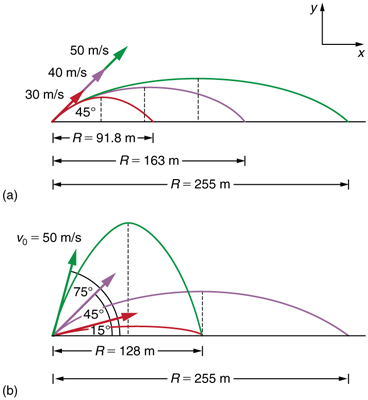Figure 5. Trajectories of projectiles on level ground. (a) The greater the initial speed v0, the greater the range for a given initial angle. (b) The effect of initial angle θ0 on the range of a projectile with a given initial speed. Note that the range is the same for 15º and 75º, although the maximum heights of those paths are different.

How does the initial velocity of a projectile affect its range? Obviously, the greater the initial speed v0, the greater the range, as shown in Figure 5(a). The initial angle θ0 also has a dramatic effect on the range, as illustrated in Figure 5(b). For a fixed initial speed, such as might be produced by a cannon, the maximum range is obtained with θ= 45º. This is true only for conditions neglecting air resistance. If air resistance is considered, the maximum angle is approximately 38º. Interestingly, for every initial angle except 45º, there are two angles that give the same range—the sum of those angles is 90º. The range also depends on the value of the acceleration of gravity g. The lunar astronaut Alan Shepherd was able to drive a golf ball a great distance on the Moon because gravity is weaker there. The range R of a projectile on level ground for which air resistance is negligible is given by

$R=\frac{{{v}_{0}}^{2}\sin{2\theta }_{0}}{g}\\$,

where v0 is the initial speed and θ0 is the initial angle relative to the horizontal. The proof of this equation is left as an end-of-chapter problem (hints are given), but it does fit the major features of projectile range as described. When we speak of the range of a projectile on level ground, we assume that R is very small compared with the circumference of the Earth. If, however, the range is large, the Earth curves away below the projectile and acceleration of gravity changes direction along the path. The range is larger than predicted by the range equation given above because the projectile has farther to fall than it would on level ground. (See Figure 6.) If the initial speed is great enough, the projectile goes into orbit. This is called escape velocity. This possibility was recognized centuries before it could be accomplished. When an object is in orbit, the Earth curves away from underneath the object at the same rate as it falls. The object thus falls continuously but never hits the surface. These and other aspects of orbital motion, such as the rotation of the Earth, will be covered analytically and in greater depth later in this text. Once again we see that thinking about one topic, such as the range of a projectile, can lead us to others, such as the Earth orbits. In Addition of Velocities, we will examine the addition of velocities, which is another important aspect of two-dimensional kinematics and will also yield insights beyond the immediate topic.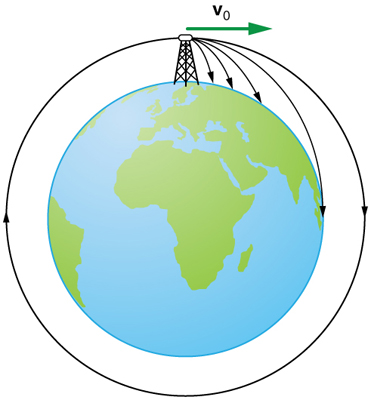Figure 6. Projectile to satellite. In each case shown here, a projectile is launched from a very high tower to avoid air resistance. With increasing initial speed, the range increases and becomes longer than it would be on level ground because the Earth curves away underneath its path. With a large enough initial speed, orbit is achieved.

PhET Explorations: Projectile Motion

Blast a Buick out of a cannon! Learn about projectile motion by firing various objects. Set the angle, initial speed, and mass. Add air resistance. Make a game out of this simulation by trying to hit a target.

Section Summary

• Projectile motion is the motion of an object through the air that is subject only to the acceleration of gravity.
• To solve projectile motion problems, perform the following steps:

1. Determine a coordinate system. Then, resolve the position and/or velocity of the object in the horizontal and vertical components. The components of position s are given by the quantities x and y, and the components of the velocity v are given by vx = v cos θ and vy = v sin θ, where v is the magnitude of the velocity and θ is its direction.

2. Analyze the motion of the projectile in the horizontal direction using the following equations:

Horizontal Motion (ax = 0)

x = x0 + vxt
vv0v= velocity is a constant.

3. Analyze the motion of the projectile in the vertical direction using the following equations:

Vertical Motion (assuming positive is up ay = -g = -9.8 m/s2)

$y={y}_{0}+\frac{1}{2}\left({v}_{0y}+{v}_{y}\right)t\\$
${v}_{y}={v}_{0y}-\text{gt}\\$
$y={y}_{0}+{v}_{0y}t-\frac{1}{2}{\mathrm{gt}}^{2}\\$
${{v}_{y}}^{2}={{v}_{0y}}^{2}-2g\left(y-{y}_{0}\right)\\$.

4. Recombine the horizontal and vertical components of location and/or velocity using the following equations:

$s=\sqrt{{x}^{2}+{y}^{2}}\\$
θ = tan−1 (y/x)
$v=\sqrt{{{v}_{x}}^{2}+{{v}_{y}}^{2}}\\$
θ= tan−1 (vy/vx).
• The maximum height h of a projectile launched with initial vertical velocity v0y is given by
$h=\frac{{{v}_{0y}}^{2}}{2g}\\$.
• The maximum horizontal distance traveled by a projectile is called the range. The range R of a projectile on level ground launched at an angle θ0 above the horizontal with initial speed v0 is given by $R=\frac{{{{v}_{0}}^{2}}\text{\sin}{2\theta }_{0}}{g}\\$.

Conceptual Questions

1. Answer the following questions for projectile motion on level ground assuming negligible air resistance (the initial angle being neither 0º nor 90º): (a) Is the velocity ever zero? (b) When is the velocity a minimum? A maximum? (c) Can the velocity ever be the same as the initial velocity at a time other than at t = 0? (d) Can the speed ever be the same as the initial speed at a time other than at t = 0?

2. Answer the following questions for projectile motion on level ground assuming negligible air resistance (the initial angle being neither 0º nor 90º): (a) Is the acceleration ever zero? (b) Is the acceleration ever in the same direction as a component of velocity? (c) Is the acceleration ever opposite in direction to a component of velocity?

3. For a fixed initial speed, the range of a projectile is determined by the angle at which it is fired. For all but the maximum, there are two angles that give the same range. Considering factors that might affect the ability of an archer to hit a target, such as wind, explain why the smaller angle (closer to the horizontal) is preferable. When would it be necessary for the archer to use the larger angle? Why does the punter in a football game use the higher trajectory?

4. During a lecture demonstration, a professor places two coins on the edge of a table. She then flicks one of the coins horizontally off the table, simultaneously nudging the other over the edge. Describe the subsequent motion of the two coins, in particular discussing whether they hit the floor at the same time.

Problems & Exercises

1. A projectile is launched at ground level with an initial speed of 50.0 m/s at an angle of 30.0º above the horizontal. It strikes a target above the ground 3.00 seconds later. What are the x and y distances from where the projectile was launched to where it lands?

2. A ball is kicked with an initial velocity of 16 m/s in the horizontal direction and 12 m/s in the vertical direction. (a) At what speed does the ball hit the ground? (b) For how long does the ball remain in the air? (c)What maximum height is attained by the ball?

3. A ball is thrown horizontally from the top of a 60.0-m building and lands 100.0 m from the base of the building. Ignore air resistance. (a) How long is the ball in the air? (b) What must have been the initial horizontal component of the velocity? (c) What is the vertical component of the velocity just before the ball hits the ground? (d) What is the velocity (including both the horizontal and vertical components) of the ball just before it hits the ground?

4. (a) A daredevil is attempting to jump his motorcycle over a line of buses parked end to end by driving up a 32º ramp at a speed of 40.0 m/s (144 km/h). How many buses can he clear if the top of the takeoff ramp is at the same height as the bus tops and the buses are 20.0 m long? (b) Discuss what your answer implies about the margin of error in this act—that is, consider how much greater the range is than the horizontal distance he must travel to miss the end of the last bus. (Neglect air resistance.)

5. An archer shoots an arrow at a 75.0 m distant target; the bull’s-eye of the target is at same height as the release height of the arrow. (a) At what angle must the arrow be released to hit the bull’s-eye if its initial speed is 35.0 m/s? In this part of the problem, explicitly show how you follow the steps involved in solving projectile motion problems. (b) There is a large tree halfway between the archer and the target with an overhanging horizontal branch 3.50 m above the release height of the arrow. Will the arrow go over or under the branch?

6. A rugby player passes the ball 7.00 m across the field, where it is caught at the same height as it left his hand. (a) At what angle was the ball thrown if its initial speed was 12.0 m/s, assuming that the smaller of the two possible angles was used? (b) What other angle gives the same range, and why would it not be used? (c) How long did this pass take?

7. Verify the ranges for the projectiles in Figure 5 (a) for θ = 45º and the given initial velocities.

8. Verify the ranges shown for the projectiles in Figure 5(b) for an initial velocity of 50 m/s at the given initial angles.

9. The cannon on a battleship can fire a shell a maximum distance of 32.0 km. (a) Calculate the initial velocity of the shell. (b) What maximum height does it reach? (At its highest, the shell is above 60% of the atmosphere—but air resistance is not really negligible as assumed to make this problem easier.) (c) The ocean is not flat, because the Earth is curved. Assume that the radius of the Earth is 6.37 × 103. How many meters lower will its surface be 32.0 km from the ship along a horizontal line parallel to the surface at the ship? Does your answer imply that error introduced by the assumption of a flat Earth in projectile motion is significant here?

10. An arrow is shot from a height of 1.5 m toward a cliff of height H. It is shot with a velocity of 30 m/s at an angle of 60º above the horizontal. It lands on the top edge of the cliff 4.0 s later. (a) What is the height of the cliff? (b) What is the maximum height reached by the arrow along its trajectory? (c) What is the arrow’s impact speed just before hitting the cliff?

11. In the standing broad jump, one squats and then pushes off with the legs to see how far one can jump. Suppose the extension of the legs from the crouch position is 0.600 m and the acceleration achieved from this position is 1.25 times the acceleration due to gravity, g. How far can they jump? State your assumptions. (Increased range can be achieved by swinging the arms in the direction of the jump.)

12. The world long jump record is 8.95 m (Mike Powell, USA, 1991). Treated as a projectile, what is the maximum range obtainable by a person if he has a take-off speed of 9.5 m/s? State your assumptions.

13. Serving at a speed of 170 km/h, a tennis player hits the ball at a height of 2.5 m and an angle θ below the horizontal. The service line is 11.9 m from the net, which is 0.91 m high. What is the angle θ such that the ball just crosses the net? Will the ball land in the service box, whose out line is 6.40 m from the net?

14. A football quarterback is moving straight backward at a speed of 2.00 m/s when he throws a pass to a player 18.0 m straight downfield. (a) If the ball is thrown at an angle of 25º relative to the ground and is caught at the same height as it is released, what is its initial speed relative to the ground? (b) How long does it take to get to the receiver? (c) What is its maximum height above its point of release?

15. Gun sights are adjusted to aim high to compensate for the effect of gravity, effectively making the gun accurate only for a specific range. (a) If a gun is sighted to hit targets that are at the same height as the gun and 100.0 m away, how low will the bullet hit if aimed directly at a target 150.0 m away? The muzzle velocity of the bullet is 275 m/s. (b) Discuss qualitatively how a larger muzzle velocity would affect this problem and what would be the effect of air resistance.

16. An eagle is flying horizontally at a speed of 3.00 m/s when the fish in her talons wiggles loose and falls into the lake 5.00 m below. Calculate the velocity of the fish relative to the water when it hits the water.

17. An owl is carrying a mouse to the chicks in its nest. Its position at that time is 4.00 m west and 12.0 m above the center of the 30.0 cm diameter nest. The owl is flying east at 3.50 m/s at an angle 30.0º below the horizontal when it accidentally drops the mouse. Is the owl lucky enough to have the mouse hit the nest? To answer this question, calculate the horizontal position of the mouse when it has fallen 12.0 m.

18. Suppose a soccer player kicks the ball from a distance 30 m toward the goal. Find the initial speed of the ball if it just passes over the goal, 2.4 m above the ground, given the initial direction to be 40º above the horizontal.

19. Can a goalkeeper at her/ his goal kick a soccer ball into the opponent’s goal without the ball touching the ground? The distance will be about 95 m. A goalkeeper can give the ball a speed of 30 m/s.

20. The free throw line in basketball is 4.57 m (15 ft) from the basket, which is 3.05 m (10 ft) above the floor. A player standing on the free throw line throws the ball with an initial speed of 7.15 m/s, releasing it at a height of 2.44 m (8 ft) above the floor. At what angle above the horizontal must the ball be thrown to exactly hit the basket? Note that most players will use a large initial angle rather than a flat shot because it allows for a larger margin of error. Explicitly show how you follow the steps involved in solving projectile motion problems.

21. In 2007, Michael Carter (U.S.) set a world record in the shot put with a throw of 24.77 m. What was the initial speed of the shot if he released it at a height of 2.10 m and threw it at an angle of 38.0º above the horizontal? (Although the maximum distance for a projectile on level ground is achieved at 45º  when air resistance is neglected, the actual angle to achieve maximum range is smaller; thus, 38º  will give a longer range than 45º  in the shot put.)

22. A basketball player is running at 5.00 m/s directly toward the basket when he jumps into the air to dunk the ball. He maintains his horizontal velocity. (a) What vertical velocity does he need to rise 0.750 m above the floor? (b) How far from the basket (measured in the horizontal direction) must he start his jump to reach his maximum height at the same time as he reaches the basket?

23. A football player punts the ball at a 45º angle. Without an effect from the wind, the ball would travel 60.0 m horizontally. (a) What is the initial speed of the ball? (b) When the ball is near its maximum height it experiences a brief gust of wind that reduces its horizontal velocity by 1.50 m/s. What distance does the ball travel horizontally?

24. Prove that the trajectory of a projectile is parabolic, having the form $y=\text{ax}+{\text{bx}}^{2}\\$. To obtain this expression, solve the equation $x={v}_{0x}t\\$ for and substitute it into the expression for $y={v}_{0y}t-\left(1/2\right){\text{gt}}^{2}\\$. (These equations describe the x and y positions of a projectile that starts at the origin.) You should obtain an equation of the form $y=\text{ax}+{\text{bx}}^{2}\\$ where a and b are constants.

25. Derive $R=\frac{{{v}_{0}}^{2}\text{\sin}{2\theta }_{0}}{g}\\$ for the range of a projectile on level ground by finding the time t at which y becomes zero and substituting this value of t into the expression for x – x0, noting that R = x – x0.

26. Unreasonable Results (a) Find the maximum range of a super cannon that has a muzzle velocity of 4.0 km/s. (b) What is unreasonable about the range you found? (c) Is the premise unreasonable or is the available equation inapplicable? Explain your answer. (d) If such a muzzle velocity could be obtained, discuss the effects of air resistance, thinning air with altitude, and the curvature of the Earth on the range of the super cannon.

27. Construct Your Own Problem Consider a ball tossed over a fence. Construct a problem in which you calculate the ball’s needed initial velocity to just clear the fence. Among the things to determine are; the height of the fence, the distance to the fence from the point of release of the ball, and the height at which the ball is released. You should also consider whether it is possible to choose the initial speed for the ball and just calculate the angle at which it is thrown. Also examine the possibility of multiple solutions given the distances and heights you have chosen.

Glossary

air resistance:
a frictional force that slows the motion of objects as they travel through the air; when solving basic physics problems, air resistance is assumed to be zero
kinematics:
the study of motion without regard to mass or force
motion:
displacement of an object as a function of time
projectile:
an object that travels through the air and experiences only acceleration due to gravity
projectile motion:
the motion of an object that is subject only to the acceleration of gravity
range:
the maximum horizontal distance that a projectile travels
trajectory:
the path of a projectile through the air

Selected Solutions to Problems & Exercises

1.  x = 1.30 m × 102, y = 30.9 m

3. (a) 3.50 s (b) 28.6 m/s (c) 34.3 m/s (d) 44.7 m/s, 50.2º below horizontal

5. (a) 18.4º (b) The arrow will go over the branch.

7. $R=\frac{{{{v}_{0}}}^{}}{\sin{2\theta }_{0}g}\\$

For θ = 45º, $R=\frac{{{{v}_{0}}}^{2}}{g}\\$

R = 91.9 m for v= 30 m/s; R = 163 m for v0; R = 255 m for v0 = 50 m/s

9. (a) 560 m/s (b) 800 × 103 m (c) 80.0 m. This error is not significant because it is only 1% of the answer in part (b).

11. 1.50 m, assuming launch angle of 45º

13. θ =6.1º. Yes, the ball lands at 5.3 m from the net

15. (a) −0.486 m (b) The larger the muzzle velocity, the smaller the deviation in the vertical direction, because the time of flight would be smaller. Air resistance would have the effect of decreasing the time of flight, therefore increasing the vertical deviation.

17. 4.23 m. No, the owl is not lucky; he misses the nest.

19. No, the maximum range (neglecting air resistance) is about 92 m.

21. 15.0 m/s

23. (a) 24.2 m/s (b) The ball travels a total of 57.4 m with the brief gust of wind.

25. $y-{y}_{0}=0={v}_{0y}t-\frac{1}{2}{gt}^{2}=\left({v}_{0}\sin\theta\right)t-\frac{1}{2}{gt}^{2}\\$ ,

so that $t=\frac{2\left({v}_{0}\sin\theta \right)}{g}\\$

$x-{x}_{0}={v}_{0x}t=\left({v}_{0}\cos\theta \right)t=R\\$, and substituting for t gives:

$R={v}_{0}\cos\theta \left(\frac{{2v}_{0}\sin\theta}{g}\right)=\frac{{{2v}_{0}}^{2}\sin\theta \cos\theta }{g}\\$

since $2\sin\theta \cos\theta =\sin 2\theta\\$, the range is:

$R=\frac{{{v}_{0}}^{2}\sin 2\theta }{g}\\$.